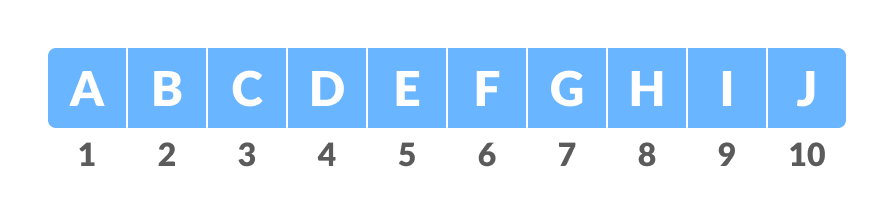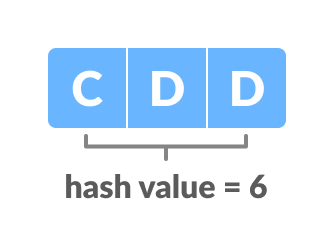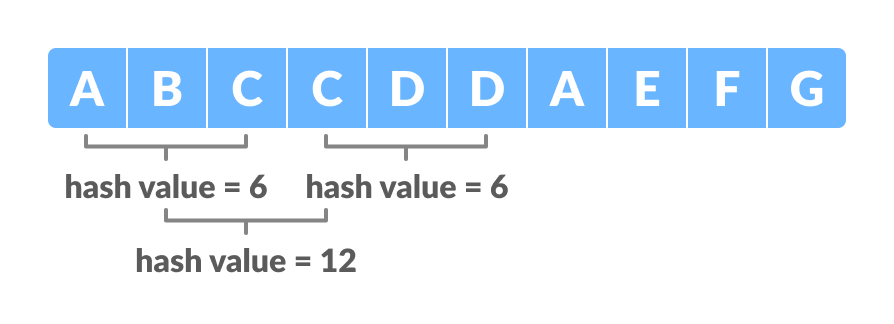# Rabin-Karp Algorithm

In this tutorial, you will learn what rabin-karp algoroithm is. Also, you will find working examples of rabin-karp algorithm in C, C++, Java and Python.

Rabin-Karp algorithm is an algorithm used for searching/matching patterns in the text using a hash function. Unlike Naive string matching algorithm, it does not travel through every character in the initial phase rather it filters the characters that do not match and then performs the comparison.

A hash function is a tool to map a larger input value to a smaller output value. This output value is called the hash value.

## How Rabin-Karp Algorithm Works?

A sequence of characters is taken and checked for the possibility of the presence of the required string. If the possibility is found then, character matching is performed.

Let us understand the algorithm with the following steps:

1. Let the text be:Text
And the string to be searched in the above text be:Pattern
2. Let us assign a `numerical value(v)/weight` for the characters we will be using in the problem. Here, we have taken first ten alphabets only (i.e. A to J).Text Weights
3. m be the length of the pattern and n be the length of the text. Here, `m = 10 and n = 3.`
Let d be the number of characters in the input set. Here, we have taken input set {A, B, C, ..., J}. So, `d = 10`. You can assume any suitable value for d.
4. Let us calculate the hash value of the pattern.Hash value of text
```hash value for pattern(p) = Σ(v * dm-1) mod 13
= ((3 * 102) + (4 * 101) + (4 * 100)) mod 13
= 344 mod 13
= 6```

In the calculation above, choose a prime number (here, 13) in such a way that we can perform all the calculations with single-precision arithmetic.

The reason for calculating the modulus is given below.

1. Calculate the hash value for the text-window of size m.
```For the first window ABC,
hash value for text(t) = Σ(v * dn-1) mod 13
= ((1 * 102) + (2 * 101) + (3 * 100)) mod 13
= 123 mod 13
= 6```
1. Compare the hash value of the pattern with the hash value of the text. If they match then, character-matching is performed.
In the above examples, the hash value of the first window (i.e. t) matches with p so, go for character matching between ABC and CDD. Since they do not match so, go for the next window.
2. We calculate the hash value of the next window by subtracting the first term and adding the next term as shown below.
```t = ((1 * 102) + ((2 * 101) + (3 * 100)) * 10 + (3 * 100)) mod 13
= 233 mod 13
= 12```

In order to optimize this process, we make use of the previous hash value in the following way.

```t = ((d * (t - v[character to be removed] * h) + v[character to be added] ) mod 13
= ((10 * (6 - 1 * 9) + 3 )mod 13
= 12
Where, h = dm-1 = 103-1 = 100.```
1. For BCC, t = 12 (6). Therefore, go for the next window.
After a few searches, we will get the match for the window CDA in the text.Hash value of different windows

## Algorithm

```n = t.length
m = p.length
h = dm-1 mod q
p = 0
t0 = 0
for i = 1 to m
p = (dp + p[i]) mod q
t0 = (dt0 + t[i]) mod q
for s = 0 to n - m
if p = ts
if p[1.....m] = t[s + 1..... s + m]
print "pattern found at position" s
If s < n-m
ts + 1 = (d (ts - t[s + 1]h) + t[s + m + 1]) mod q```

## Python, Java and C/C++ Examples

``````# Rabin-Karp algorithm in python

d = 10

def search(pattern, text, q):
m = len(pattern)
n = len(text)
p = 0
t = 0
h = 1
i = 0
j = 0

for i in range(m-1):
h = (h*d) % q

# Calculate hash value for pattern and text
for i in range(m):
p = (d*p + ord(pattern[i])) % q
t = (d*t + ord(text[i])) % q

# Find the match
for i in range(n-m+1):
if p == t:
for j in range(m):
if text[i+j] != pattern[j]:
break

j += 1
if j == m:
print("Pattern is found at position: " + str(i+1))

if i < n-m:
t = (d*(t-ord(text[i])*h) + ord(text[i+m])) % q

if t < 0:
t = t+q

text = "ABCCDDAEFG"
pattern = "CDD"
q = 13
search(pattern, text, q)``````
``````// Rabin-Karp algorithm in Java

public class RabinKarp {
public final static int d = 10;

static void search(String pattern, String txt, int q) {
int m = pattern.length();
int n = txt.length();
int i, j;
int p = 0;
int t = 0;
int h = 1;

for (i = 0; i < m - 1; i++)
h = (h * d) % q;

// Calculate hash value for pattern and text
for (i = 0; i < m; i++) {
p = (d * p + pattern.charAt(i)) % q;
t = (d * t + txt.charAt(i)) % q;
}

// Find the match
for (i = 0; i <= n - m; i++) {
if (p == t) {
for (j = 0; j < m; j++) {
if (txt.charAt(i + j) != pattern.charAt(j))
break;
}

if (j == m)
System.out.println("Pattern is found at position: " + (i + 1));
}

if (i < n - m) {
t = (d * (t - txt.charAt(i) * h) + txt.charAt(i + m)) % q;
if (t < 0)
t = (t + q);
}
}
}

public static void main(String[] args) {
String txt = "ABCCDDAEFG";
String pattern = "CDD";
int q = 13;
search(pattern, txt, q);
}
}``````
``````// Rabin-Karp algorithm in C

#include <stdio.h>
#include <string.h>

#define d 10

void rabinKarp(char pattern[], char text[], int q) {
int m = strlen(pattern);
int n = strlen(text);
int i, j;
int p = 0;
int t = 0;
int h = 1;

for (i = 0; i < m - 1; i++)
h = (h * d) % q;

// Calculate hash value for pattern and text
for (i = 0; i < m; i++) {
p = (d * p + pattern[i]) % q;
t = (d * t + text[i]) % q;
}

// Find the match
for (i = 0; i <= n - m; i++) {
if (p == t) {
for (j = 0; j < m; j++) {
if (text[i + j] != pattern[j])
break;
}

if (j == m)
printf("Pattern is found at position:  %d \n", i + 1);
}

if (i < n - m) {
t = (d * (t - text[i] * h) + text[i + m]) % q;

if (t < 0)
t = (t + q);
}
}
}

int main() {
char text[] = "ABCCDDAEFG";
char pattern[] = "CDD";
int q = 13;
rabinKarp(pattern, text, q);
}``````
``````// Rabin-Karp algorithm in C++

#include <string.h>

#include <iostream>
using namespace std;

#define d 10

void rabinKarp(char pattern[], char text[], int q) {
int m = strlen(pattern);
int n = strlen(text);
int i, j;
int p = 0;
int t = 0;
int h = 1;

for (i = 0; i < m - 1; i++)
h = (h * d) % q;

// Calculate hash value for pattern and text
for (i = 0; i < m; i++) {
p = (d * p + pattern[i]) % q;
t = (d * t + text[i]) % q;
}

// Find the match
for (i = 0; i <= n - m; i++) {
if (p == t) {
for (j = 0; j < m; j++) {
if (text[i + j] != pattern[j])
break;
}

if (j == m)
cout << "Pattern is found at position: " << i + 1 << endl;
}

if (i < n - m) {
t = (d * (t - text[i] * h) + text[i + m]) % q;

if (t < 0)
t = (t + q);
}
}
}

int main() {
char text[] = "ABCCDDAEFG";
char pattern[] = "CDD";
int q = 13;
rabinKarp(pattern, text, q);
}``````

## Limitations of Rabin-Karp Algorithm

### Spurious Hit

When the hash value of the pattern matches with the hash value of a window of the text but the window is not the actual pattern then it is called a spurious hit.

Spurious hit increases the time complexity of the algorithm. In order to minimize spurious hit, we use modulus. It greatly reduces the spurious hit.

## Rabin-Karp Algorithm Complexity

The average case and best case complexity of Rabin-Karp algorithm is `O(m + n)` and the worst case complexity is O(mn).

The worst-case complexity occurs when spurious hits occur a number for all the windows.

## Rabin-Karp Algorithm Applications

• For pattern matching
• For searching string in a bigger text